Transformer 是谷歌大脑在2017年底发表的论文Attention Is All You Need中所提出的 seq2seq 模型。现在已经取得了大范围的应用和扩展，而 BERT 就是从 Transformer 中衍生出来的预训练语言模型。

1. Transformer 直观认识
2. Positional Encoding
3. Self Attention Mechanism
4. 残差连接和 Layer Normalization
5. Transformer Encoder 整体结构
6. Transformer Decoder 整体结构
7. 总结
8. 参考文章

# 0. Transformer 直观认识

Transformer 和 LSTM 的最大区别，就是 LSTM 的训练是迭代的、串行的，必须要等当前字处理完，才可以处理下一个字。而 Transformer 的训练时并行的，即所有字是同时训练的，这样就大大增加了计算效率。Transformer 使用了位置嵌入 (Positional Encoding) 来理解语言的顺序，使用自注意力机制（Self Attention Mechanism）和全连接层进行计算，这些后面会讲到。

Transformer 模型主要分为两大部分，分别是 EncoderDecoderEncoder 负责把输入（语言序列）隐射成隐藏层（下图中第 2 步用九宫格代表的部分），然后解码器再把隐藏层映射为自然语言序列。例如下图机器翻译的例子（Decoder 输出的时候，是通过 N 层 Decoder Layer 才输出一个 token，并不是通过一层 Decoder Layer 就输出一个 token）。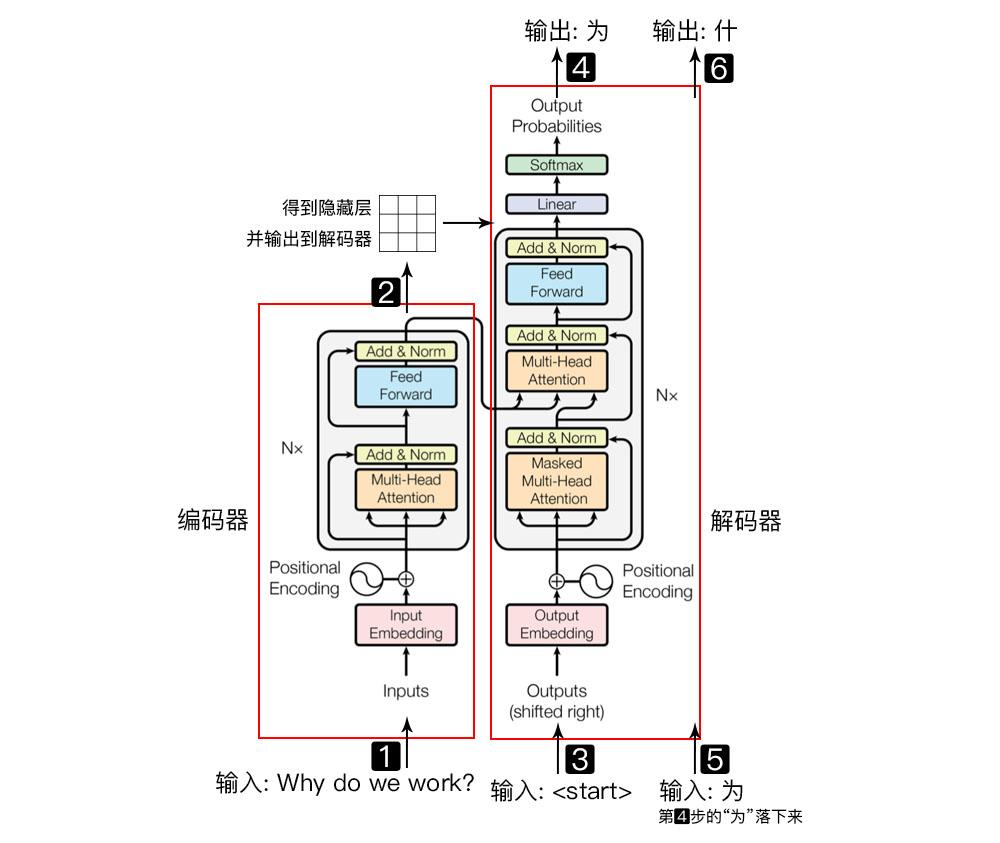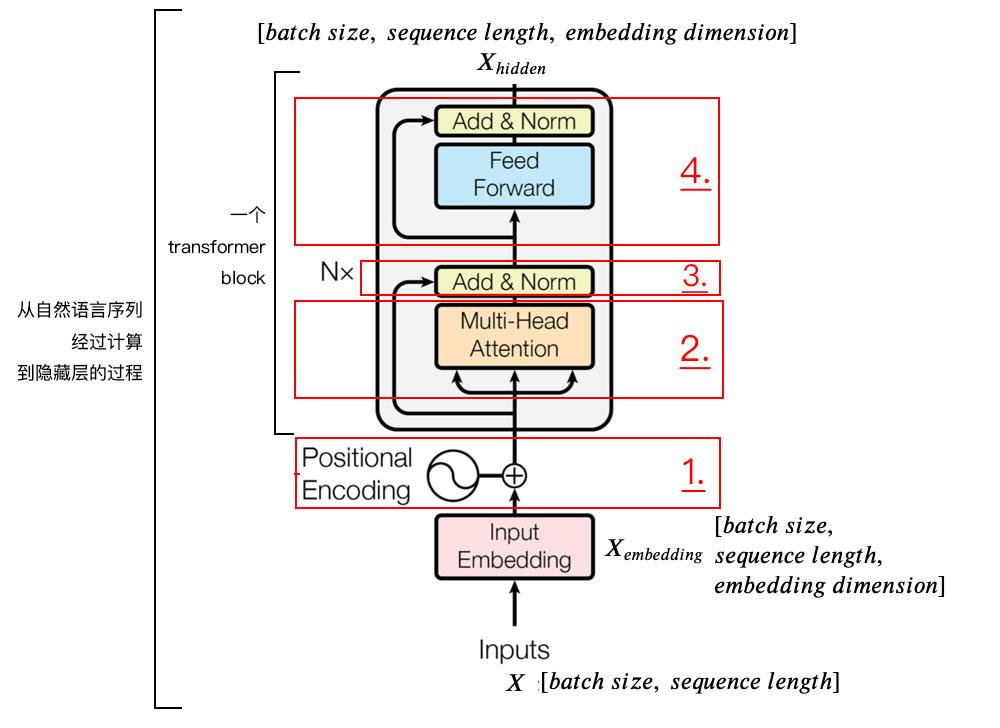# 1. Positional Encoding

\begin{array}{c}
P E(p o s, 2 i)=\sin \left(p o s / 10000^{2 i / d_{\text {model }}}\right) \\
P E(p o s, 2 i+1)=\cos \left(p o s / 10000^{2 i / d_{\text {model }}}\right)
\end{array}

import numpy as np
import matplotlib.pyplot as plt
import seaborn as sns
import math

def get_positional_encoding(max_seq_len, embed_dim):
# 初始化一个positional encoding
# embed_dim: 字嵌入的维度
# max_seq_len: 最大的序列长度
positional_encoding = np.array([
[pos / np.power(10000, 2 * i / embed_dim) for i in range(embed_dim)]
if pos != 0 else np.zeros(embed_dim) for pos in range(max_seq_len)])

positional_encoding[1:, 0::2] = np.sin(positional_encoding[1:, 0::2])  # dim 2i 偶数
positional_encoding[1:, 1::2] = np.cos(positional_encoding[1:, 1::2])  # dim 2i+1 奇数
return positional_encoding

positional_encoding = get_positional_encoding(max_seq_len=100, embed_dim=16)
plt.figure(figsize=(10,10))
sns.heatmap(positional_encoding)
plt.title("Sinusoidal Function")
plt.xlabel("hidden dimension")
plt.ylabel("sequence length")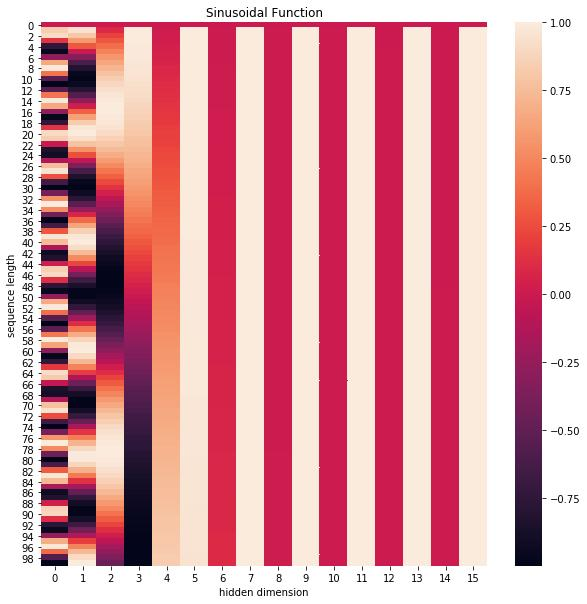plt.figure(figsize=(8, 5))
plt.plot(positional_encoding[1:, 1], label="dimension 1")
plt.plot(positional_encoding[1:, 2], label="dimension 2")
plt.plot(positional_encoding[1:, 3], label="dimension 3")
plt.legend()
plt.xlabel("Sequence length")
plt.ylabel("Period of Positional Encoding")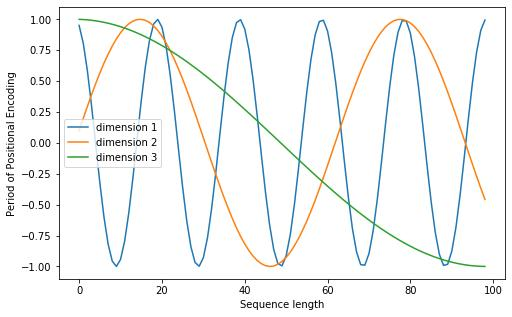# 2.Self Attention Mechanism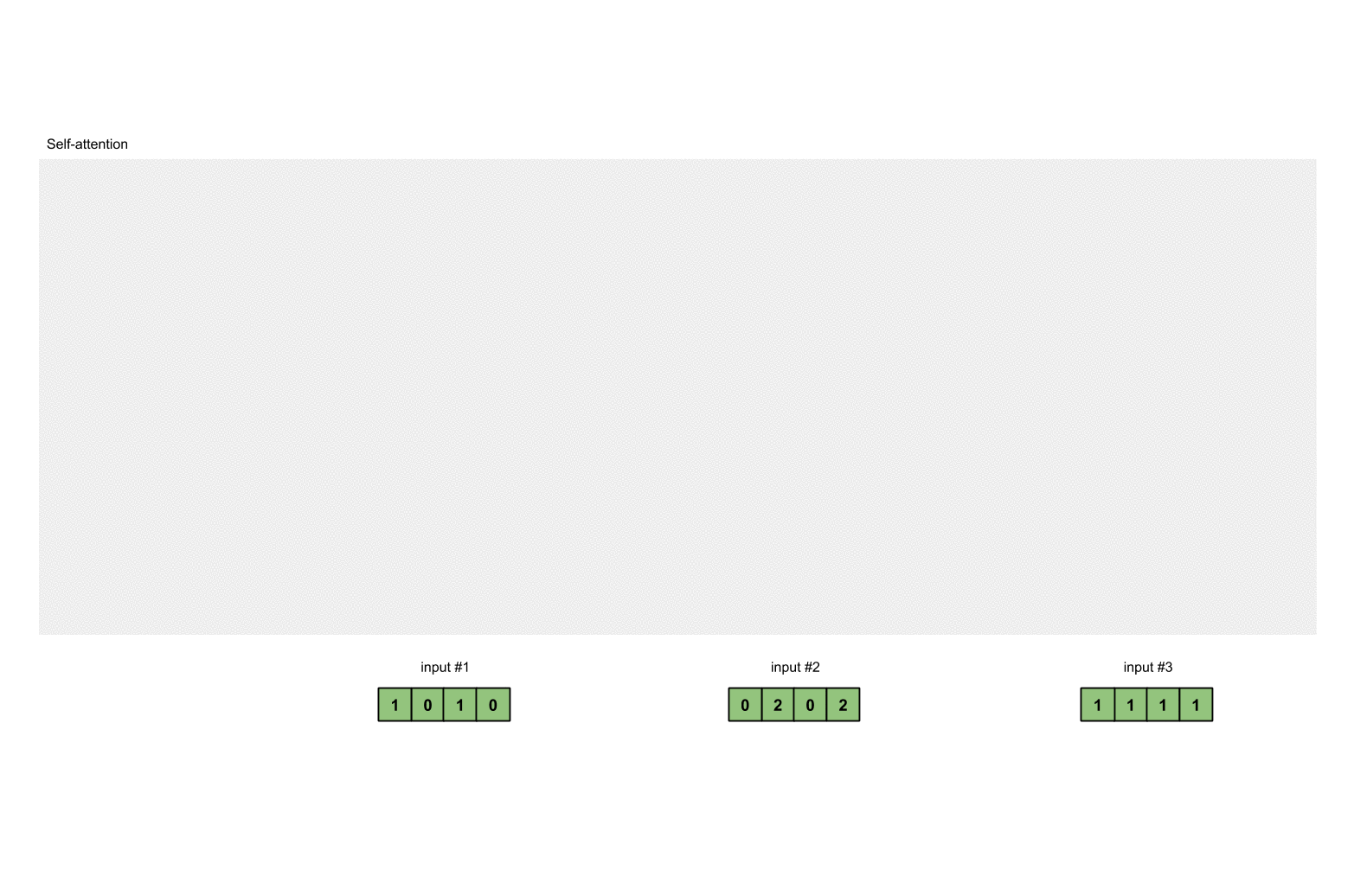[0, 4, 2]
[1, 0, 2] x [1, 4, 3] = [2, 4, 4]
[1, 0, 1]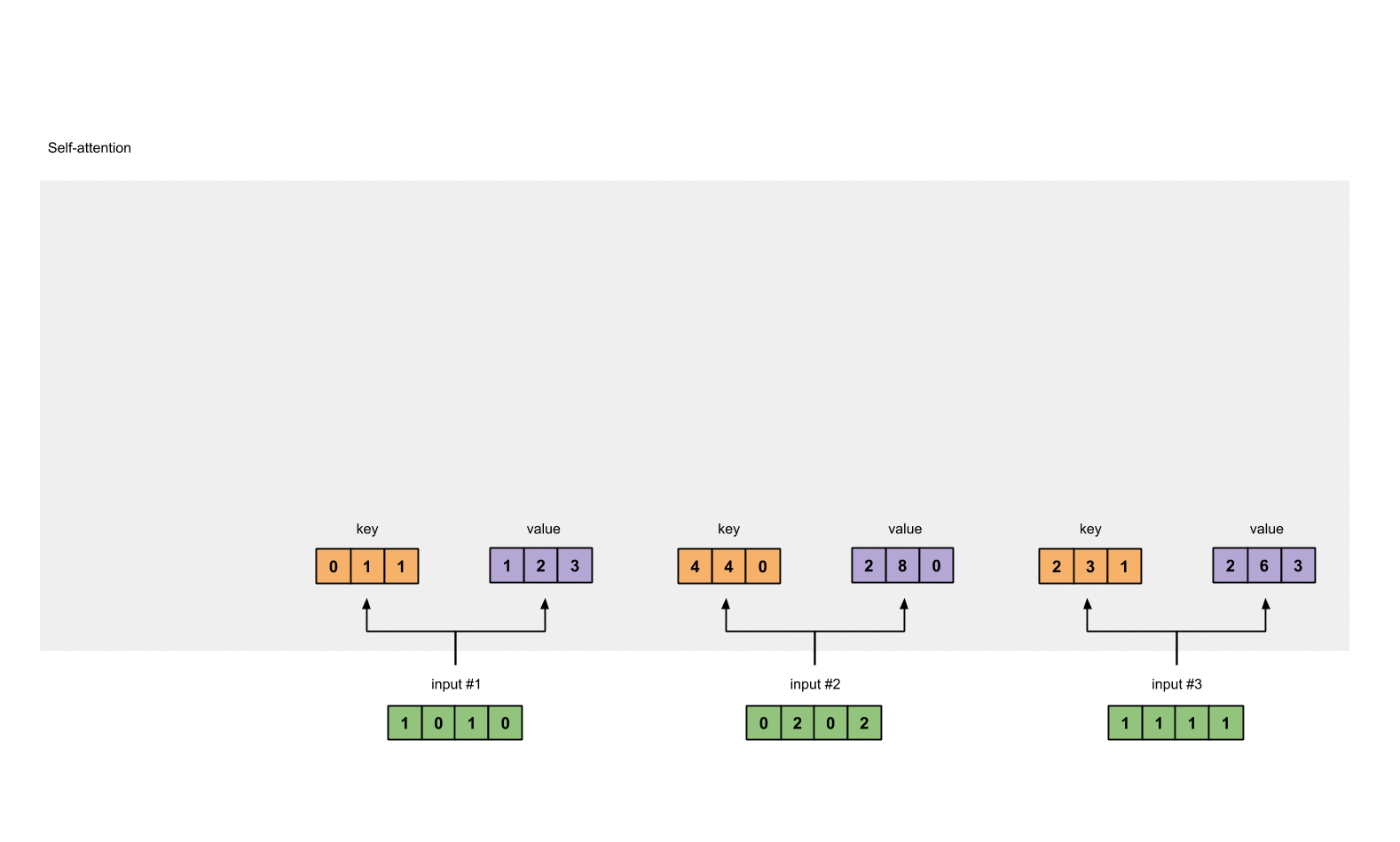softmax([2, 4, 4]) = [0.0, 0.5, 0.5]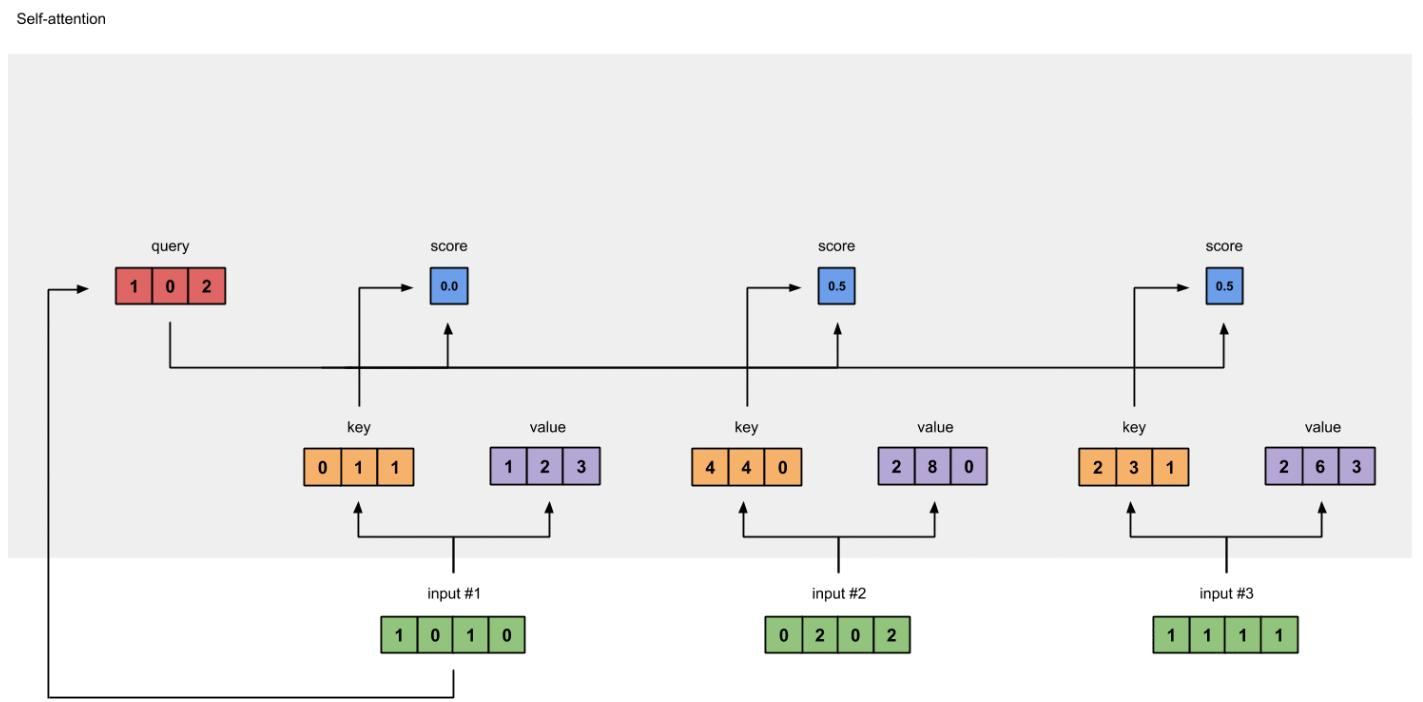0.0 * [1, 2, 3] = [0.0, 0.0, 0.0]
0.5 * [2, 8, 0] = [1.0, 4.0, 0.0]
0.5 * [2, 6, 3] = [1.0, 3.0, 1.5]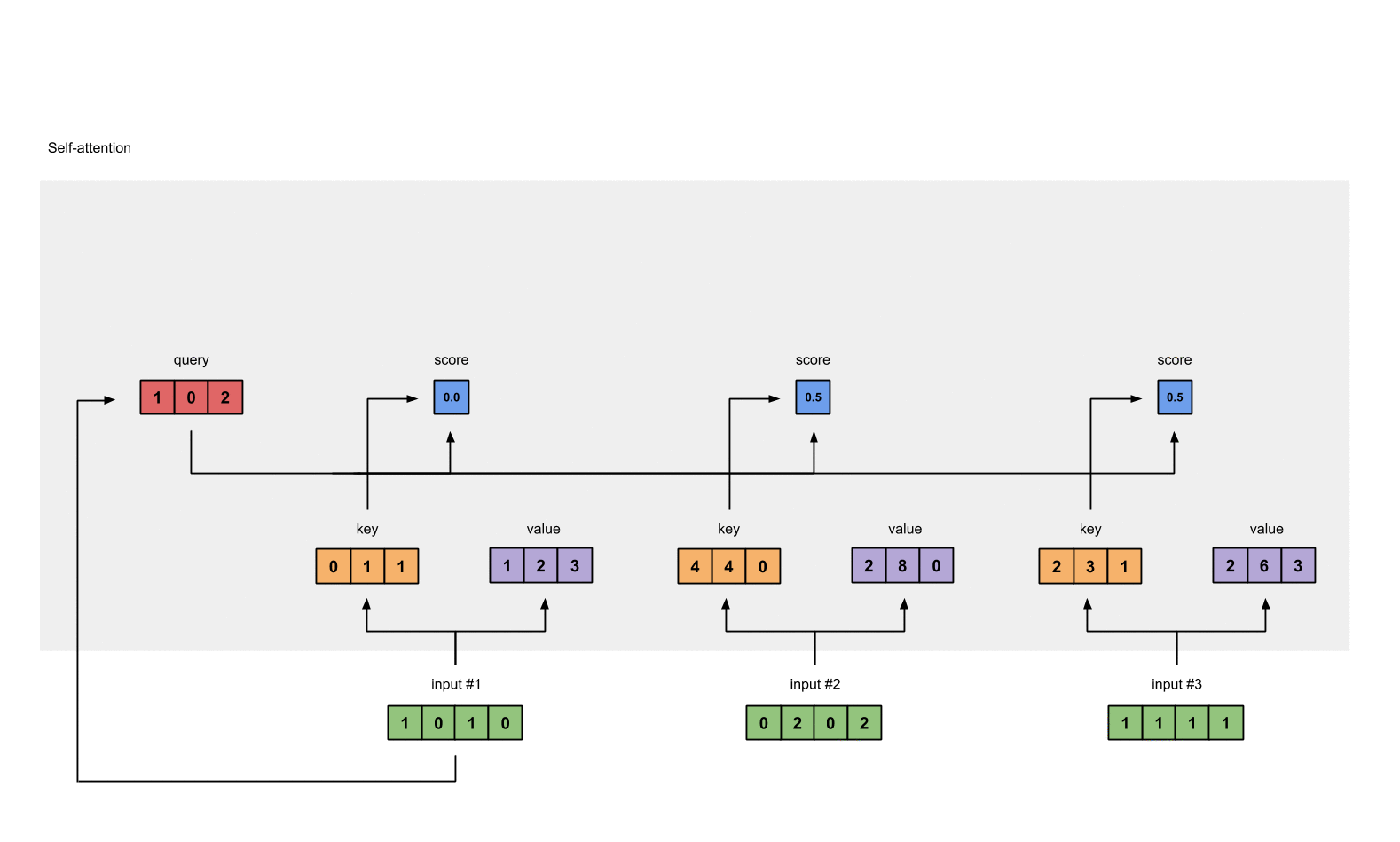[0.0, 0.0, 0.0]
+ [1.0, 4.0, 0.0]
+ [1.0, 3.0, 1.5]
-----------------
= [2.0, 7.0, 1.5]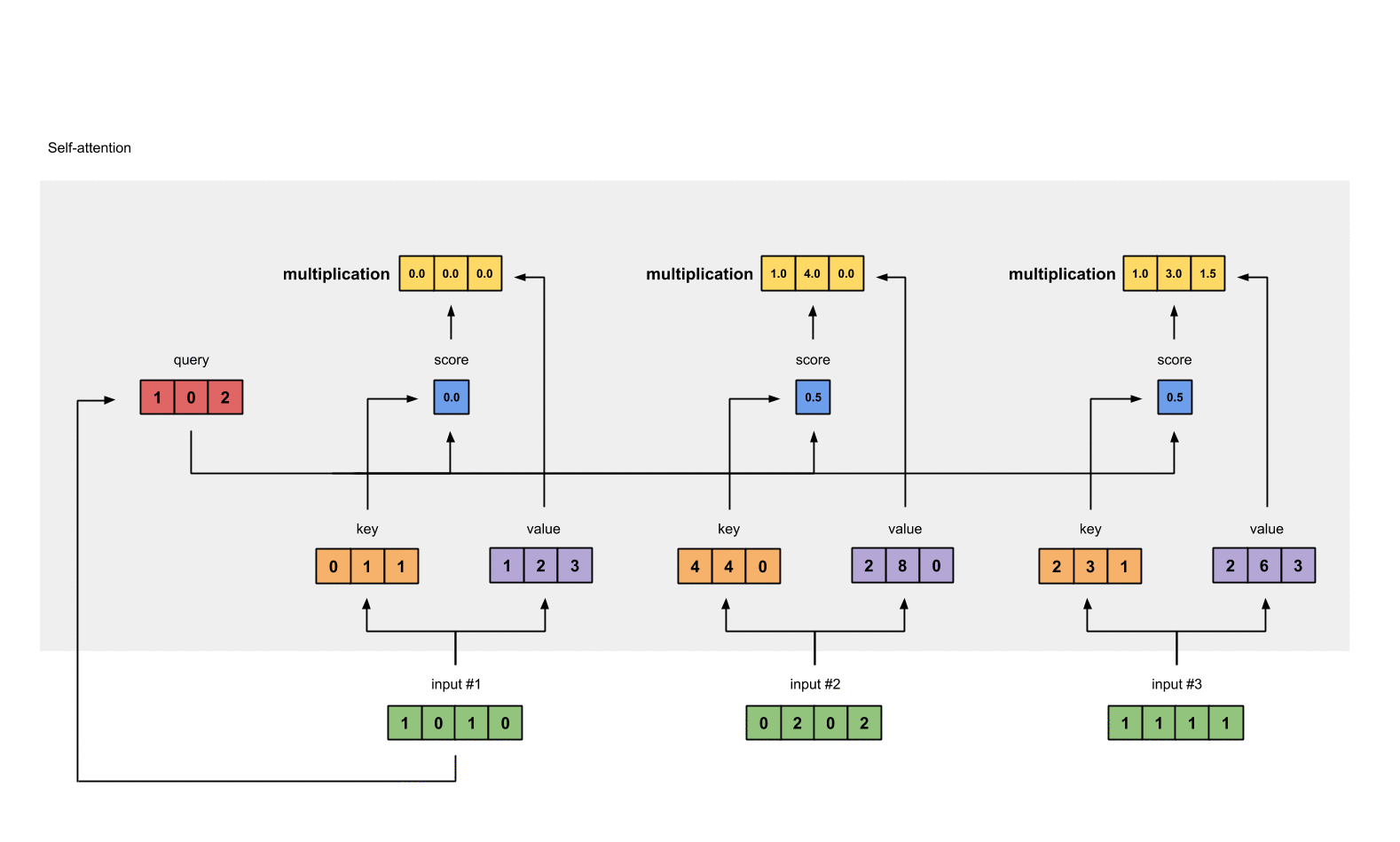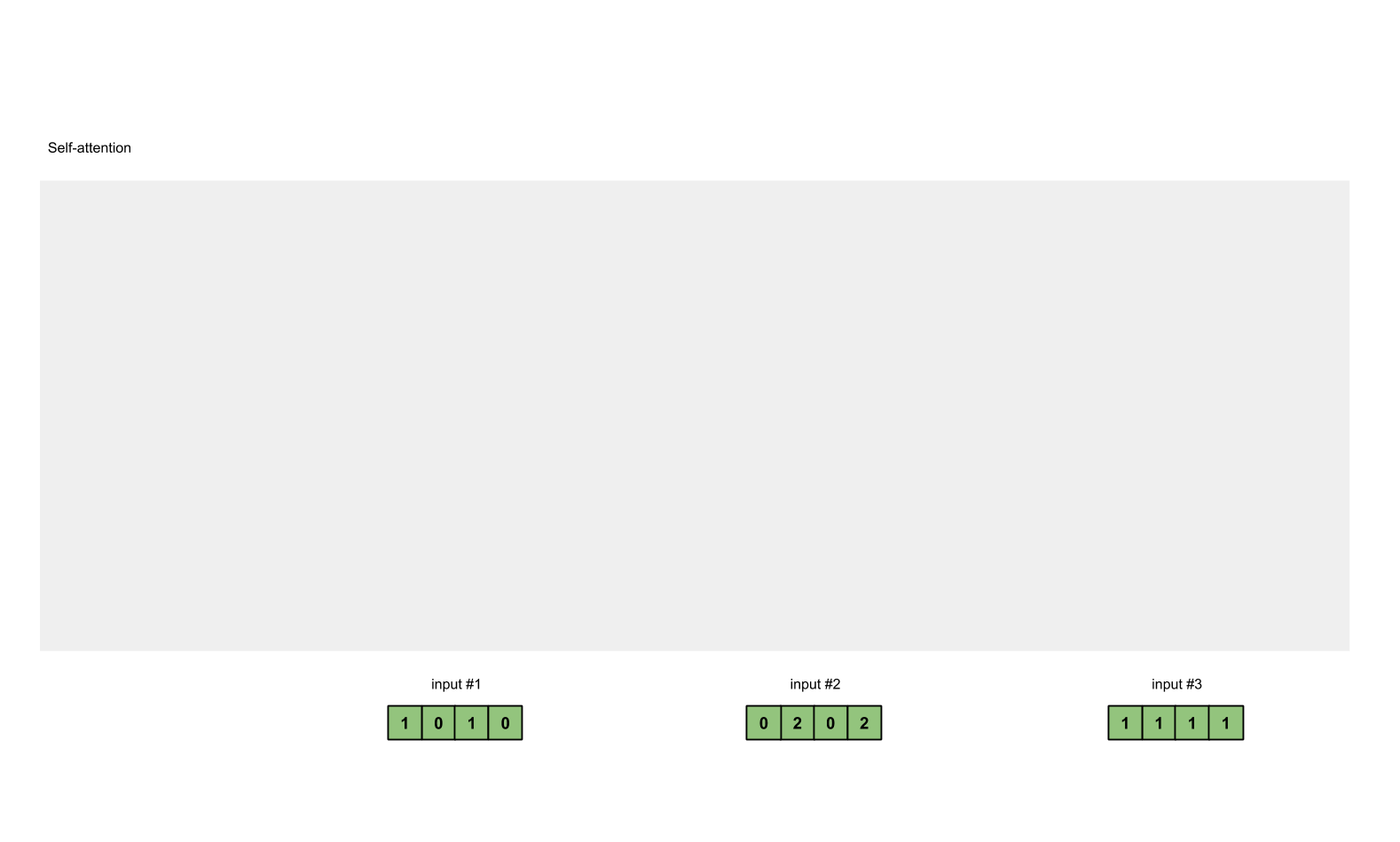## 矩阵计算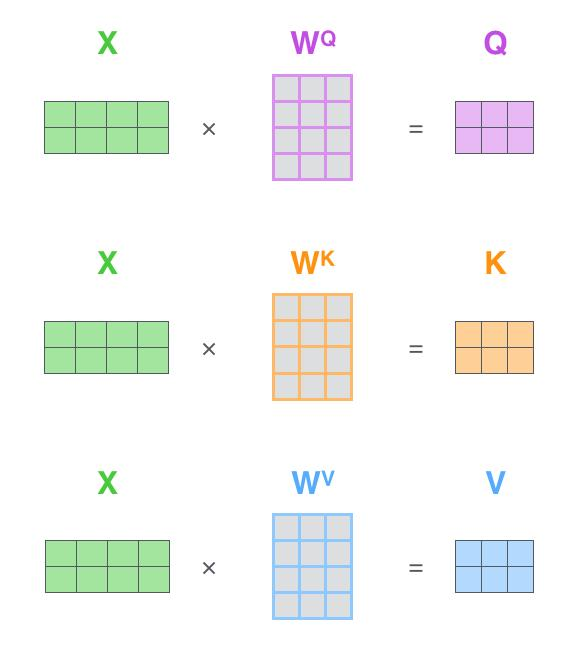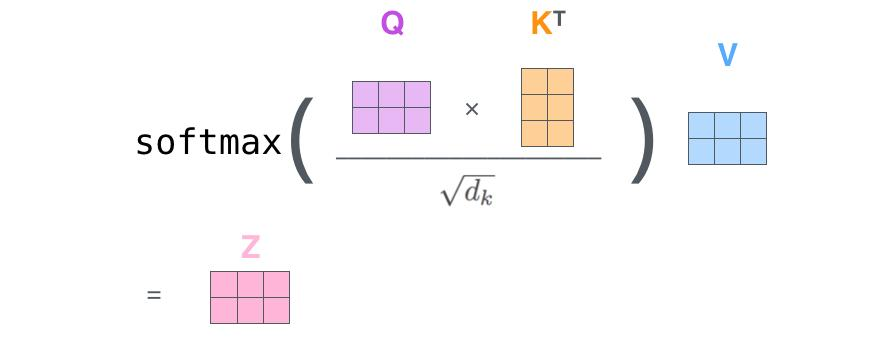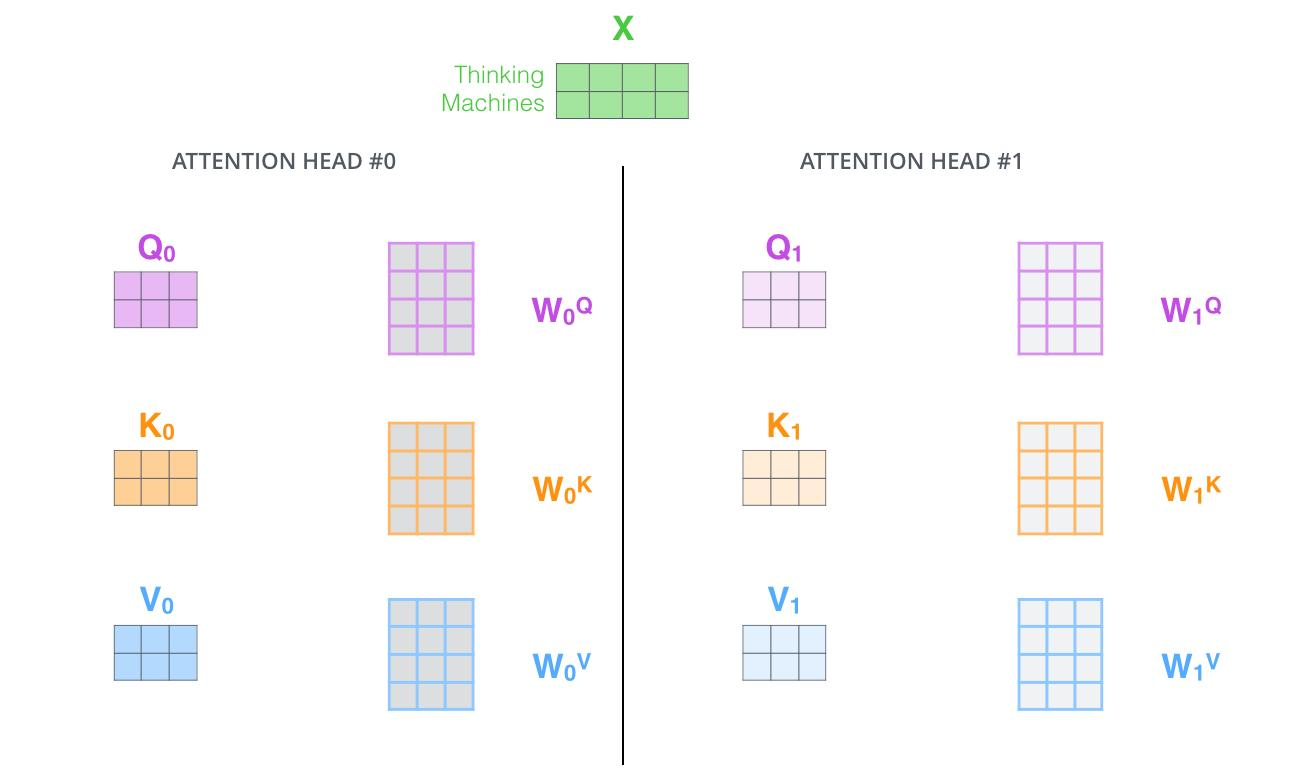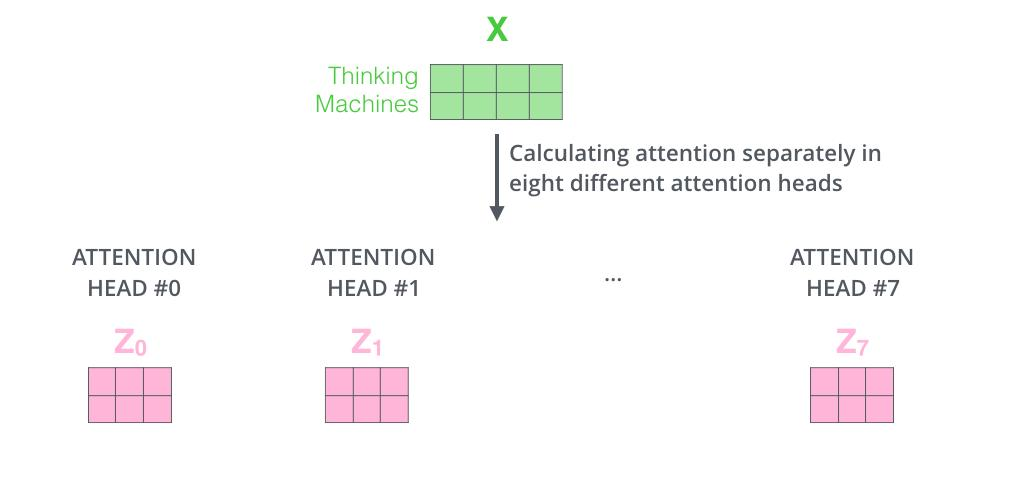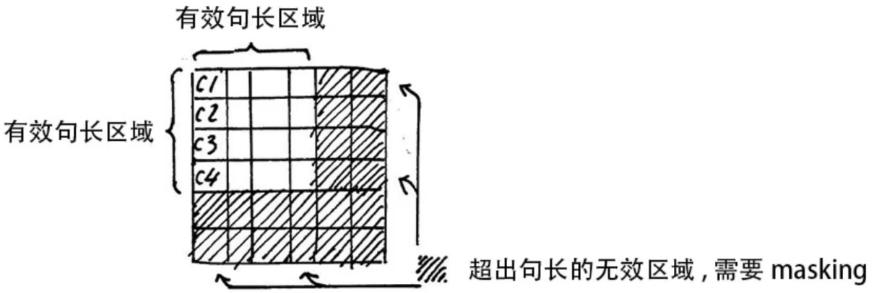\sigma (z_{i})=\frac{e^{z_{i}} }{ {\textstyle \sum_{j=1}^{K}}e^{z_{j}} }

\begin{array}{c}
Z_{illegal}=Z_{illegal}+bias_{illegal} \\
bias_{illegal}\longrightarrow -\infty
\end{array}

# 3. 残差连接和 Layer Normalization

## 残差连接

x_{embedding}+\text {Self-Attention}(Q,K,V)

## Layer Normalization

Layer Normalization 的作用是把神经网络中隐藏层归一为标准正态分布，也就是i.i.d独立同分布，以起到加快训练速度，加速收敛的作用

\mu _{j}=\frac{1}{m} \sum_{i=1}^{m}x_{ij}

\sigma _{j}^{2} = \frac{1}{m} \sum_{i=1}^{m} (x_{ij}-\mu _{j})^{2}

LayerNormal(x)=\frac{x_{ij}-\mu _{j}}{\sqrt{\sigma _{j}^{2}+\epsilon } }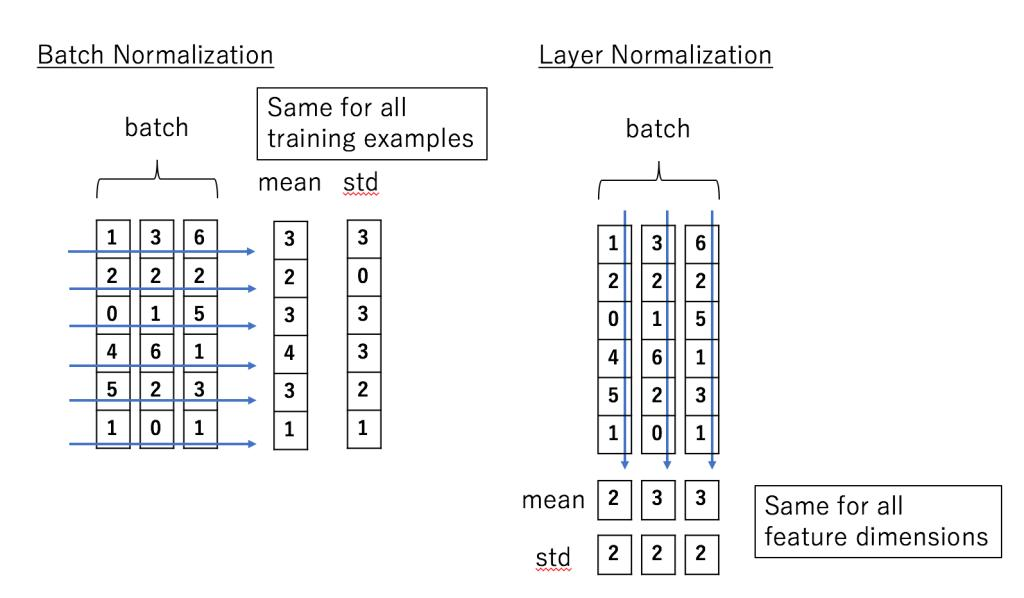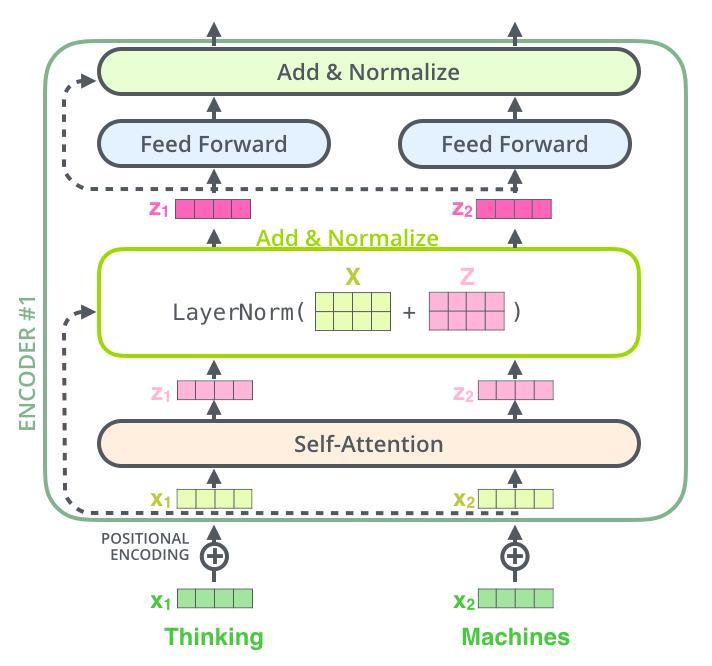# 4. Transformer Encoder 整体结构

(1) 字向量与位置编码

X=\text { Embedding-Lookup }(X)+\text { Positional-Encoding }

(2) 自注意力机制

\begin{array}{c}
Q = Linear_{q}(X) = XW_{Q} \\
K = Linear_{k}(X) = XW_{K} \\
V = Linear_{v}(X) = XW_{V} \\
X_{attention} = \text{Self-Attention}(Q,K,V)
\end{array}

(3) self-attention 残差连接与 Layer Normalization

\begin{array}{c}
X_{attention} = X + X_{attention} \\
X_{attention} = LayerNorm(X_{attention})
\end{array}

(4) 下面进行 Encoder block 结构图中的第 4 部分，也就是 FeedForward，其实就是两层线性映射并用激活函数激活，比如说ReLU

X_{hidden} = Linear(ReLU(Linear(X_{attention})))

(5) FeedForward 残差连接与 Layer Normalization

\begin{array}{c}
X_{hidden} = X_{attention} + X_{hidden} \\
X_{hidden} = LayerNorm(X_{hidden})
\end{array}

X_{hidden} \in \mathbb{R}^{\text {batchsize * seqlen. * embeddim }}

# 5. Transformer Decoder 整体结构

• Multi-Head Encoder-Decoder Attention
• FeedForward Network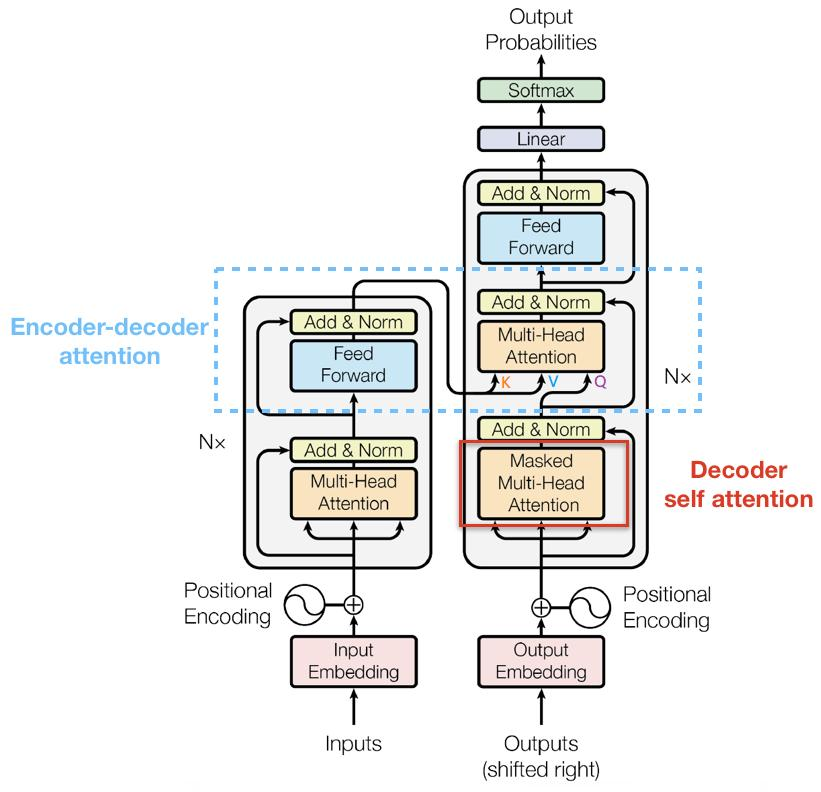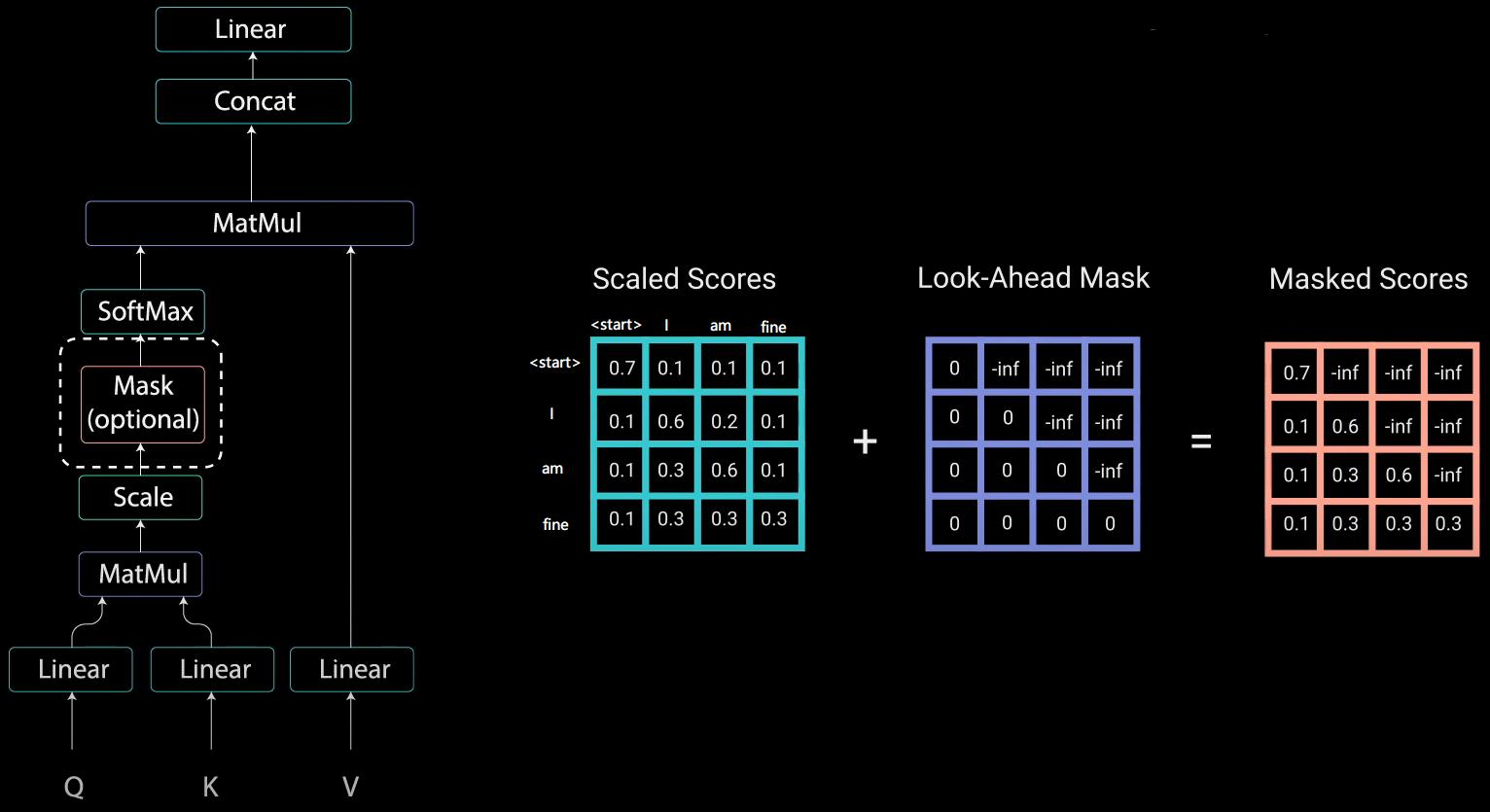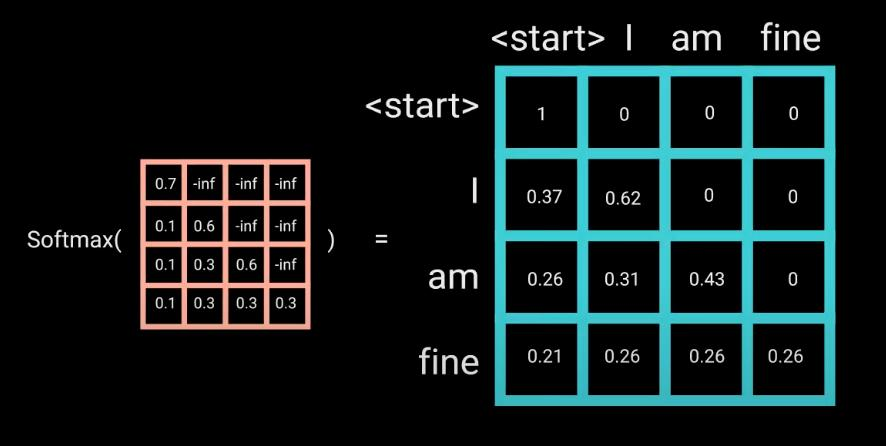Multi-Head Self-Attention 无非就是并行的对上述步骤多做几次，前面 Encoder 也介绍了，这里就不多赘述了

## Masked Encoder-Decoder Attention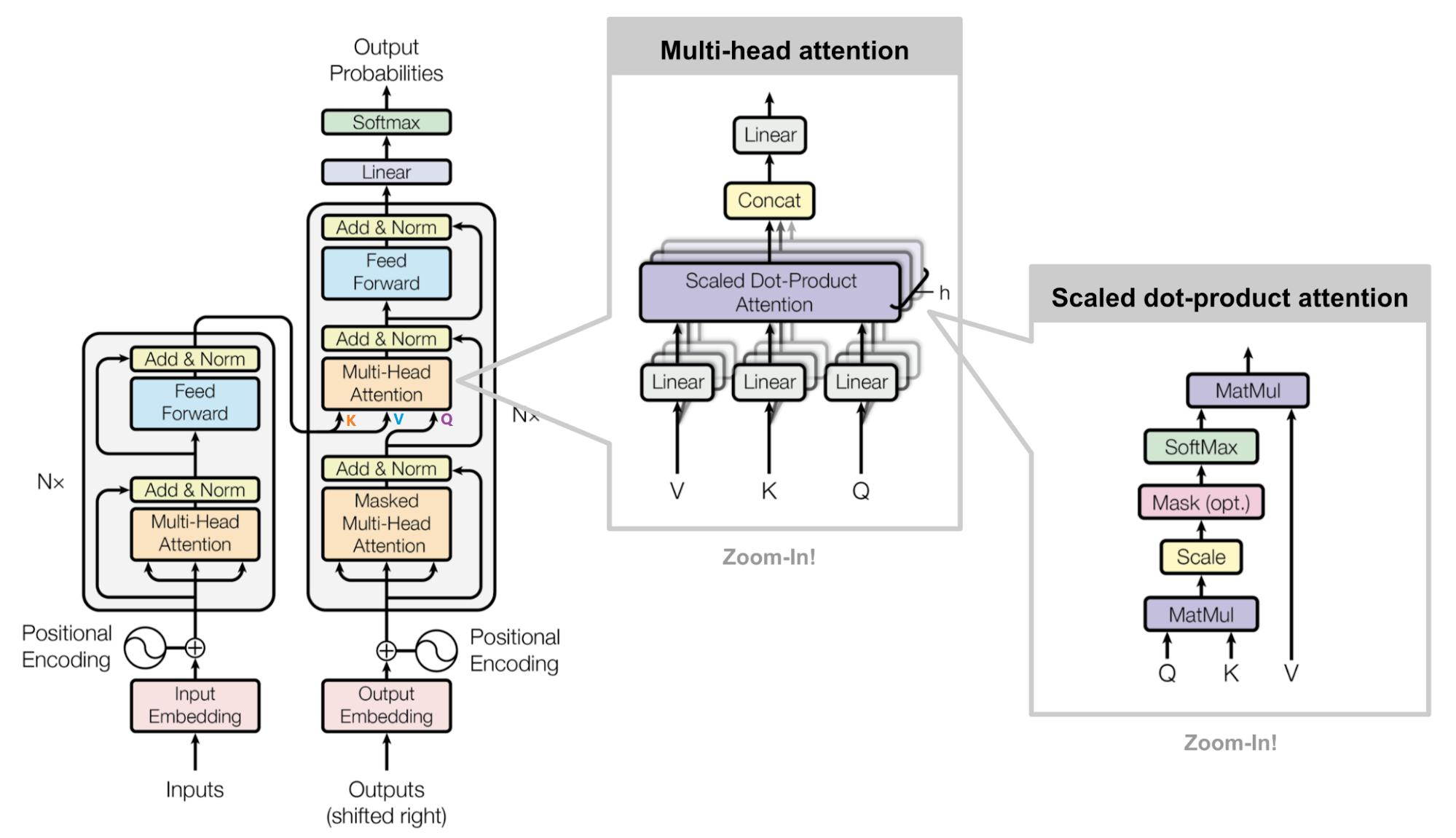# 6 总结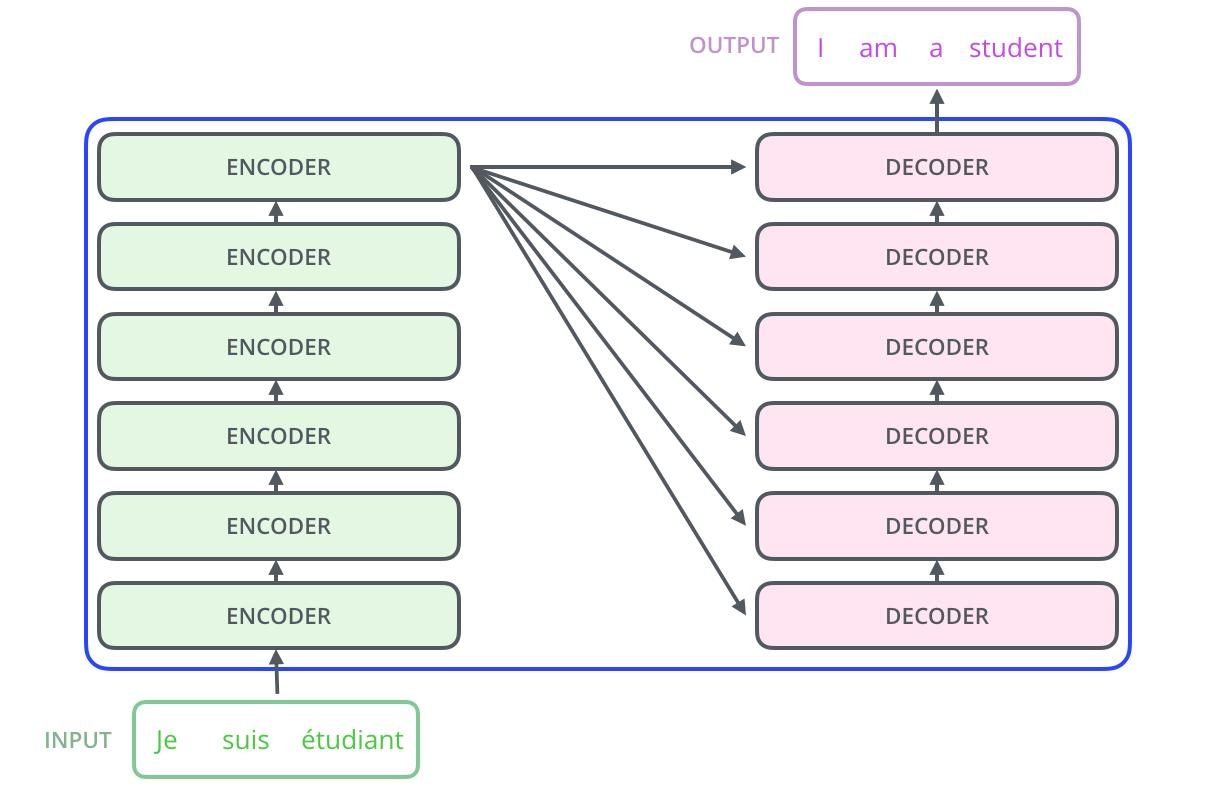## Transformer 相比于 RNN/LSTM，有什么优势？为什么？

1. RNN 系列的模型，无法并行计算，因为 T 时刻的计算依赖 T-1 时刻的隐层计算结果，而 T-1 时刻的计算依赖 T-2 时刻的隐层计算结果
2. Transformer 的特征抽取能力比 RNN 系列的模型要好